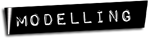# Team:USP-Brazil/Model:RFPVisibility## RFP Visual Contrast Analysis

### Stating the problem

Here is the problem: we are trying to build a “machine” to detect methanol in alcoholic drinks using a genetically modified yeast to produce RFP (Red Fluorescent Protein) through methanol consumption. The chosen yeast was Pichia pastoris, especially because its natural ability to interact with methanol due to the presence of an AOX promoter.

Among the questions we need to answer in order to accomplish this task, is the simple question about how do we “see” RFP signal that would be produced by methanol induction. To address this question we make use of some mathematical notions of visual contrast and brightness.

### Definitions

Let's define more precisely the meaning of visual contrast:

Visual Contrast: Consider some object placed at a distance x from an observer and emitting light with a certain intensity. This light is measured by the observer and called I(x). Suppose the background light intensity measured by the observer is IB(x).

We define the Visual Contrast (C) as:

Quantum Yield and Molar Extinction Coefficient: The brightness of a FP (Fluorescent Protein) is proportional to the product of its Quantum Yield and its Molar Extinction Coefficient (MEC).

The QY is defined by the rate of emitted fotons and absorbed fotons and we call it Φ.

The MEC, defined as the absorption force per mol per centimeter (M-1cm-1), is a property of the FP's chemical structure and we call it ε.

Beer-Lambert Law: Consider an object placed at x0=0 emitting light. Call I(x) the light intensity at a distance x. The Beer-Lambert law says the light intensity distant x from x0 suffers an exponential attenuation:

Where A is the Total Absorptivity of the medium where the object is placed, ci are the absorbing components and εi are their respective MECs.

### Putting it all together

Well, there is a natural link between the concepts described above. To see this we need to describe the intensity produced by the RFP through its QY and MEC, than we estimate the environment conditions and choose a desired contrast and determine the necessary concentration of RFP to achieve this.

Looking closely to the relevant quantity concerning the RFP, we can interpret the product Φε as the number of fotons absorbed and transformed in red light per mol of RFP per centimeter of distance.

In normal environment we don't need to be concerned about the frequency of interaction of a RFP molecule and a foton because there are fotons in abundance. So we can come up with a simple formula to the intensity produced by concentration of RFP in a certain container of size D.

Where IB represents the background (locally homogeneous) light intensity and [RFP] the RFP concentration. This formula states that all the background light inside the container with RFP is transformed in red light and the intensity is adjusted by the factor Φε D[RFP].

Inserting this in the definition of visual contrast

and extending the homogeneity of background light intensity we can treat IB(x)=IB for all possible values of x. This leads us to

Here A represents the atmospheric air absorptivity. And finally, the RFP concentration can be determined

### Giving an estimate

Now, we can use our knowledge about the human vision limits and about the RFP to estimate the RFP concentration necessary for it to be seen. To this end, we make two additional hypothesis and A =0.5 m-1 and x=1 m, i.e., the atmospheric air absorbs half the light it interacts per meter and the observer will measure at 1 meter from the RFP container. Our data is:

• RFP mCherry QY: Φ=0,22
• RFP mCherry MEC: ε=72000
• Visual contrast: C=0,10
• D∼2 cm

The value for D was chosen to represent a cylindrical flask with 2 cm diameter. The value for C is a mean calculated from data from . Therefore, [RFP] ∼ 8.9 × 10-5mols

### Conclusion

With these results we can compare the RFP production rate of Pichia pastoris with the photostability of our RFP to determine whether we will be able to see the RFP produced in different conditions. However, more specific data will be required to proceed.

#### References

 Contrast Thresholds of the Human Eye - H. RICHARD BLACKWELL -JOURNAL OF THE OPTICAL SOCIETY OF AMERICA VOLUME 36, NUMBER 11 NOVEMBER, 1946

RFP Visibility | Deterministic Model | Stochastic Model